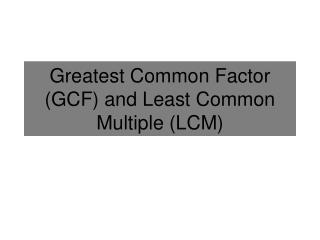DownloadDownload PresentationGreatest Common Factor (GCF) and Least Common Multiple (LCM)

# Greatest Common Factor (GCF) and Least Common Multiple (LCM)

Download Presentation## Greatest Common Factor (GCF) and Least Common Multiple (LCM)

- - - - - - - - - - - - - - - - - - - - - - - - - - - E N D - - - - - - - - - - - - - - - - - - - - - - - - - - -
##### Presentation Transcript

1. Greatest Common Factor (GCF) and Least Common Multiple (LCM)

2. Prime Factorization Prime Factorization is when a number is expressed as a product of prime factors. You can use a factor tree to find the prime factorization of a number. Example: Find the prime factorization of12. 12 2 6 2 3 2

3. Factoring Monomials You can use prime factorization to factor monomials. A monomial is in factored form when it is expressed as the product of prime numbers and variables and no variable has an exponent greater than 1. Example: Factor 100mn3. 100 mn3 = mnnn 10 10 5 2 5 2

4. Finding a GCF through Reasoning Find the greatest common factor of: 8, 28, 12 Factors of 8: Factors of 28: Factors of 12: Find the largest number that divides all three numbers. The GCF is 4. This process could be long and tedious if the numbers are large. A more efficient method is desirable.

5. Greatest Common Factor (GCF) The Greatest Common Factor (GCF) is the greatest expression that is a factor of the original expressions. Procedure to find the GCF of two or more terms: • Factor each monomial. • The GCF is the product of the common factors.

6. Examples Find the GCF of each set of monomials: • 24, 60, and 72 • 15 and 8 • 15a2b, 9ab2, and 18ab Step One: Factor each Monomial. Step Two: Find the common factors. There is nothing in common, so the GCF is… Step Three: Multiply the factors in common.

7. Finding a LCM through Reasoning Find the least common multiple of: 8, 28, 12 Multiples of 8: Multiples of 28: Multiples of 12: Find the smallest number that is a multiple of all three. The LCM is 168. This process could be long and tedious if the numbers are large. A more efficient method is desirable.

8. Least Common Multiple (LCM) The Least Common Multiple (LCM) is the least number that is a common multiple of two or more expressions. Procedure to find the LCM of two or more terms: • Factor each monomial. • Find the greatest number of times each factor appears in each factorization. • The LCM is the product of (2).

9. Examples Find the GCF of each set of monomials: • 18, 30, and 105 • 15a2b and 27b3 Step One: Factor each Monomial. Step Two: Find the greatest number of times each factor appears in each factorization. Step Three: Multiply the result of (2).